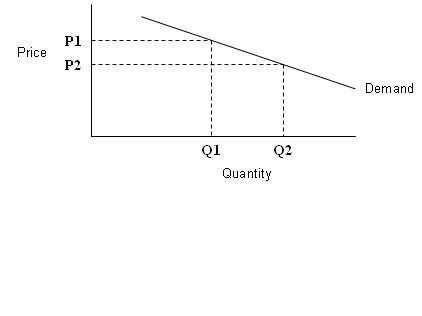# Elasticity Between Two Points

238

Elasticity Between Two Points

Elasticity can also be calculated between two points.
In this figure, elasticity from point K to L is -4.
ЄKL = percentage change in Qd
Percentage change in P
= 16– 8 ÷ 6 – 8
8 8
= – 4
Since absolute value is greater than 1 so it is elastic.
Similarly we can also calculate for inelastic demand curve.
Arc Elasticity
Arc elasticity measures the “average” elasticity between two points on the demand curve. The formula is simply (change in quantity/change in price)*(average price/average quantity).

# Unemployment in Pakistan

To measure arc elasticity we take average values for Q and P respectively.
Point Elasticity
Point elasticity is used when the change in price is very small, i.e. the two points between which elasticity is being measured essentially collapse on each other. Differential calculus is used to calculate the instantaneous rate of change of quantity with respect to changes in price (dQ/dP) and then this is multiplied by P/Q, where P and Q are the price and quantity obtaining at the point of interest. The formula for point elasticity can be illustrated as:
Є=ΔQ x P
Δ P Q
Or this formula can also be written as:
Є= dQ x P
d P Q
Where d = infinitely small change in price.
If elasticity = zero then demand curve will be vertical.
If elasticity is infinity then the demand curve will be horizontal. Virtual University